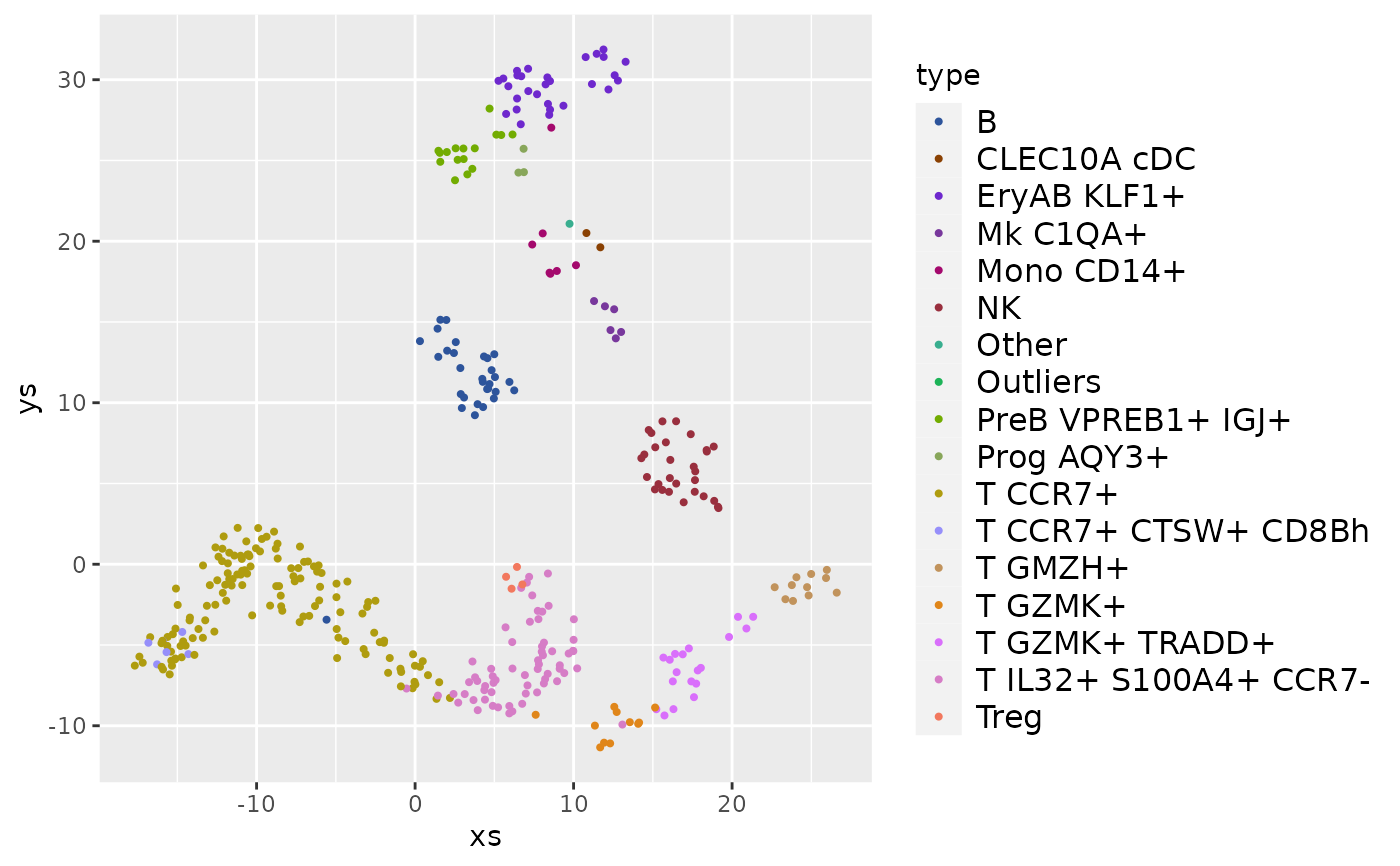This is a thin wrapper to ggplot2::discrete_scale('colour', 'chameleon', ...), which uses the colors chosen by invoking distinct_colors. The order of the colors is arbitrary. If the data has some structure the colors should reflect, use one of the many palettes available in R, or using data_colors for automatically matching the colors to the structure of multi-dimensional data.

scale_color_chameleon(
minimal_saturation = 33,
minimal_lightness = 20,
maximal_lightness = 80,
...
)

## Arguments

minimal_saturation Exclude colors whose saturation (hypot(a, b) in CIELAB color space) is less than this value (by default, 33). Exclude colors whose lightnes (l in CIELAB color space) is less than this value (by default, 20). Exclude colors whose lightnes (l in CIELAB color space) is more than this value (by default, 80). Additional parameters for discrete_scale.

## Examples

library(ggplot2)
data(pbmc)
frame <- as.data.frame(pbmc$umap) frame$type <- pbmc\$types
ggplot(frame, aes(x=xs, y=ys, color=type)) +
geom_point(size=0.75) +
scale_color_chameleon() +
theme(legend.text=element_text(size=12), legend.key.height=unit(14, 'pt'))
#> Warning: Removed 12 rows containing missing values (geom_point).